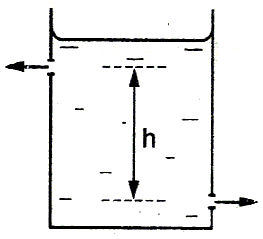# Will it move?

Fill in the blank:

There are two identical small holes on the opposite sides of a tank containing a liquid as shown below. The tank is open at the top. The difference in heights between the two holes is $h$. As the liquid comes out of the two holes, the tank will experience a net horizontal force proportional to $\text{\_\_\_\_\_\_\_\_\_\_\_}$.×